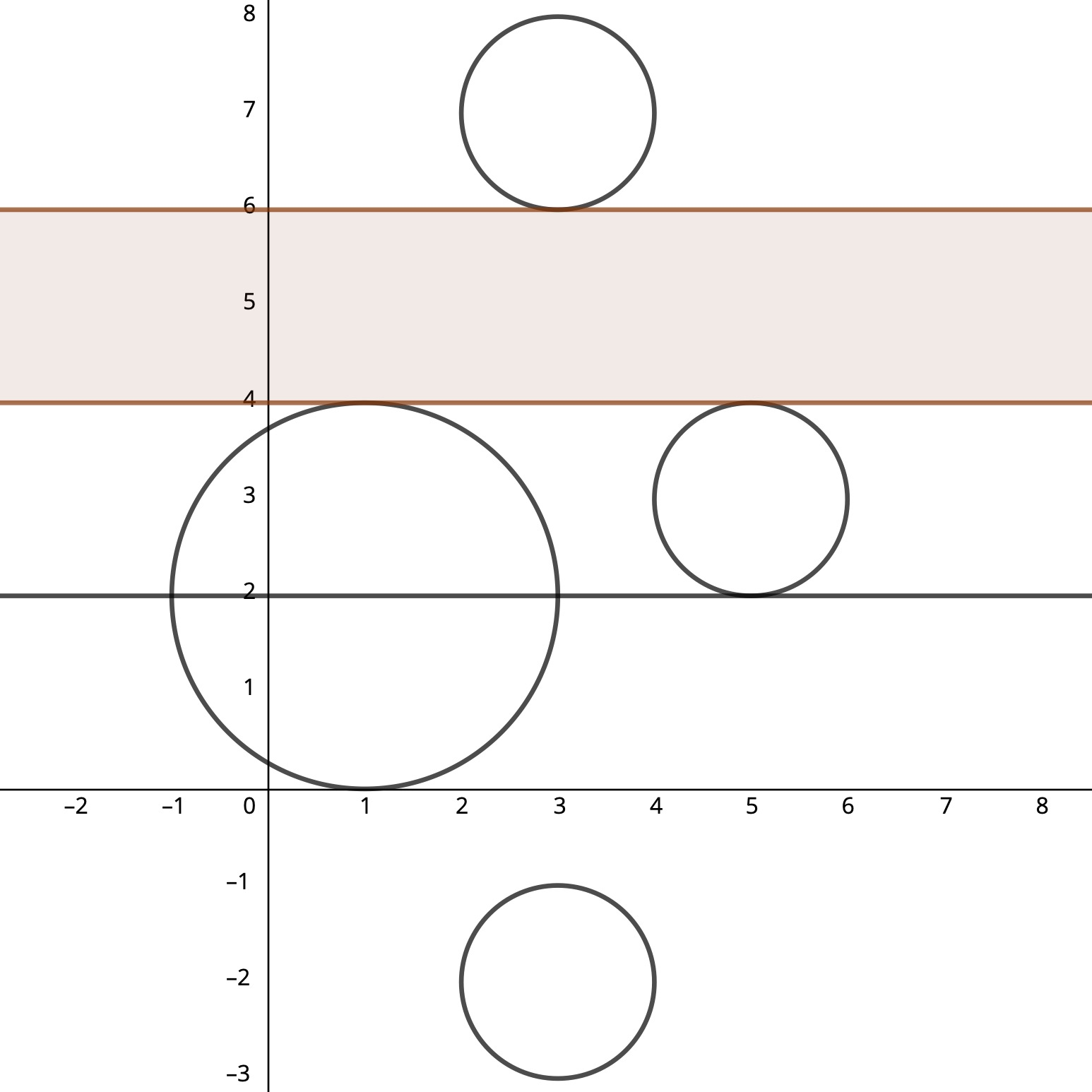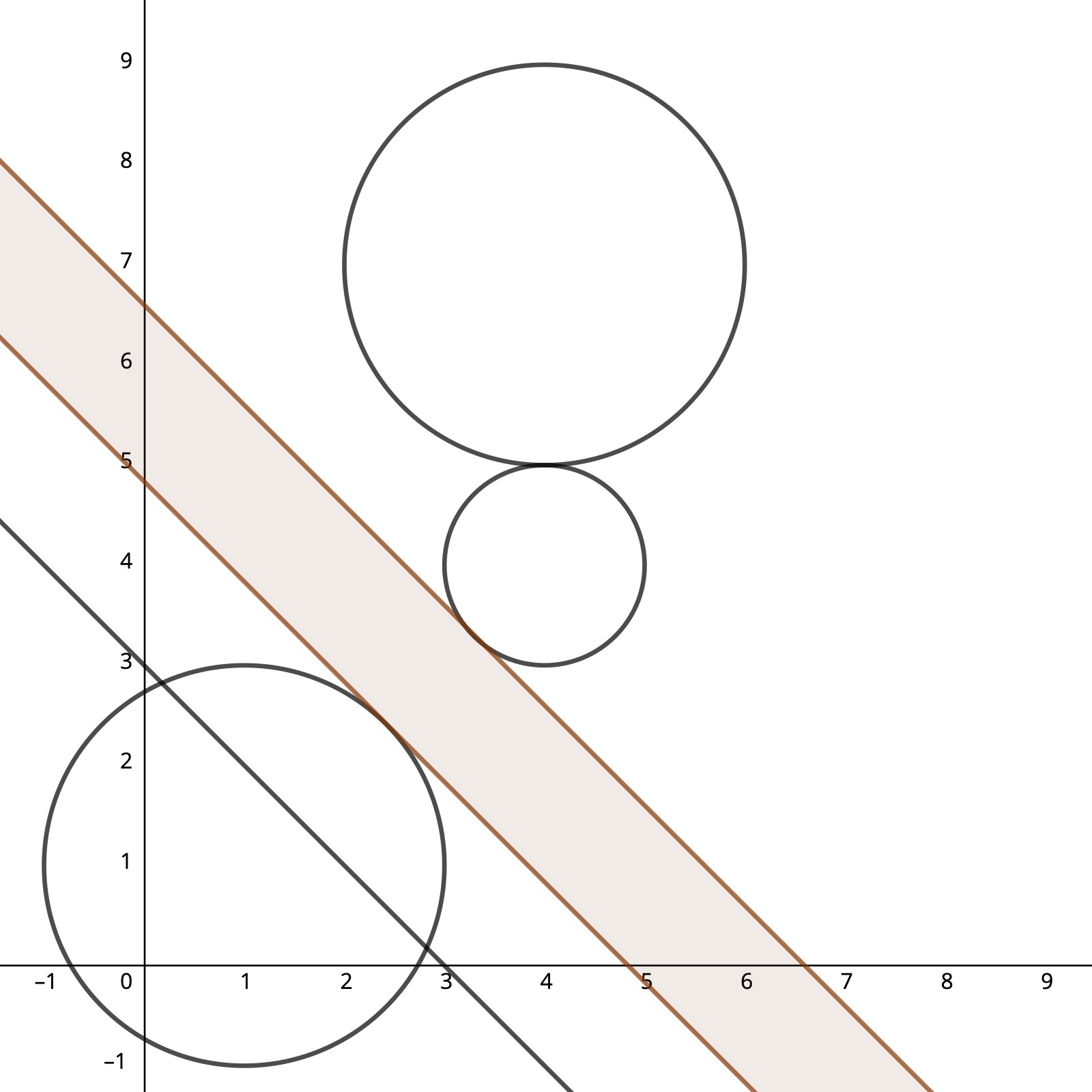시간 제한 메모리 제한 제출 정답 맞은 사람 정답 비율
2 초 512 MB 3 1 1 100.000%

## 문제

The burger eating contest is coming! The contest is taking place at two different cities. But there was a slight miscalculation --- there is no direct road between these cities across the forest. To save enough time organizers decided to build a direct road with maximum width through the forest without cutting any trees. Moreover, it was decided to leave at least one tree on both sides of the road.

For simplicity, let's represent each tree as a circle on plane with Cartesian coordinates. Build a road of infinite length and maximum width parallel to straight line, that passes through two given points.

## 입력

First line contains four integers $x_s$, $y_s$, $x_f$ and $y_f$ --- coordinates of points $(x_s, y_s)$ and $(x_f, y_f)$ that the line passes through.

Second line contains single integer $n$ --- number of trees in the forest ($2 \le n \le 2 \cdot 10^5$). There are no intersecting trees, but they can touch each other.

Each of the next $n$ lines contains three integers $x_i$, $y_i$ and $r_i$ --- coordinates of the center and radius of $i$-th tree ($1 \le r_i \le 10^9$).

All coordinates' absolute values don't exceed $10^9$.

## 출력

Output maximum width of the road parallel to straight line, that passes through points $(x_s, y_s)$ and $(x_f, y_f)$. There should be at least one tree on both sides of the road. The road may touch the trees, but not intersect them. If there is no such road, output 0.

Your answer will be accepted, if it's absolute or relative error doesn't exceed $10^{-6}$. Formally speaking, if your answer is $a$ and jury's is $b$, then it will be accepted if $\frac {|a - b|}{\max(1, b)} \le 10^{-6}$.

## 서브태스크

번호 배점 제한
1 27

$2 \le n \le 100$

2 19

All radii are equal

3 23

The straight line is parallel to axes

4 31

No additional constraints

## 예제 입력 1

0 2 4 2
4
5 3 1
3 -2 1
1 2 2
3 7 1


## 예제 출력 1

2.000000000000000


## 예제 입력 2

0 3 3 0
3
1 1 2
4 4 1
4 7 2


## 예제 출력 2

1.242640687119285


## 힌트

 First example Second example## 채점 및 기타 정보

• 예제는 채점하지 않는다.